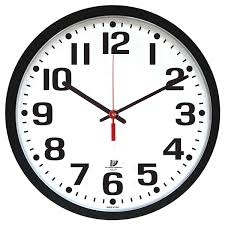# Younger 7033

Cyril was four times less than Dalibor 3 years ago. How many years will Cyril be 2x less if he is 3x younger now?

x =  9

### Step-by-step explanation:

(c-3) = (d-3)/4
c = d/3
(c+x) = (d+x)/2

4c-d = 9
3c-d = 0
2c-d+x = 0

Row 2 - 3/4 · Row 1 → Row 2
4c-d = 9
-0.25d = -6.75
2c-d+x = 0

Row 3 - 2/4 · Row 1 → Row 3
4c-d = 9
-0.25d = -6.75
-0.5d+x = -4.5

Pivot: Row 2 ↔ Row 3
4c-d = 9
-0.5d+x = -4.5
-0.25d = -6.75

Row 3 - -0.25/-0.5 · Row 2 → Row 3
4c-d = 9
-0.5d+x = -4.5
-0.5x = -4.5

x = -4.5/-0.5 = 9
d = -4.5-x/-0.5 = -4.5-9/-0.5 = 27
c = 9+d/4 = 9+27/4 = 9

c = 9
d = 27
x = 9

Our linear equations calculator calculates it.Did you find an error or inaccuracy? Feel free to write us. Thank you!

Tips for related online calculators
Do you have a system of equations and looking for calculator system of linear equations?
Do you want to convert time units like minutes to seconds?